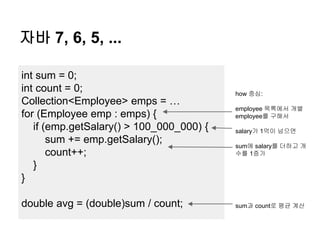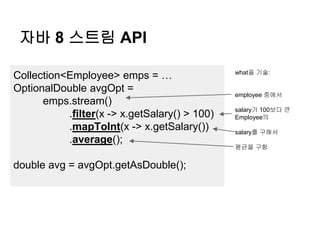Successfully reported this slideshow.

# 자바8 스트림 API 소개

92

Share×
1 of 20
1 of 20

# 자바8 스트림 API 소개

92

Share

자바8 스트림 API 소개

자바8 스트림 API 소개

## More Related Content

### Related Books

Free with a 14 day trial from Scribd

See all

### Related Audiobooks

Free with a 14 day trial from Scribd

See all

### 자바8 스트림 API 소개

1. 1. 자바8 스트림 API 소개 최범균, 2013-06-03
2. 2. 자바 7, 6, 5, ... int sum = 0; int count = 0; Collection<Employee> emps = … for (Employee emp : emps) { if (emp.getSalary() > 100_000_000) { sum += emp.getSalary(); count++; } } double avg = (double)sum / count; how 중심: employee 목록에서 개별 employee를 구해서 salary가 1억이 넘으면 sum에 salary를 더하고 개 수를 1증가 sum과 count로 평균 계산
3. 3. 자바 8 스트림 API Collection<Employee> emps = … OptionalDouble avgOpt = emps.stream() .filter(x -> x.getSalary() > 100) .mapToInt(x -> x.getSalary()) .average(); double avg = avgOpt.getAsDouble(); what을 기술: employee 중에서 salary가 100보다 큰 Employee의 salary를 구해서 평균을 구함
4. 4. 스트림 API: 세 단계 구성 emps.stream().filter(x -> x.getSalary() > 100).count(); 스트림 생성 중개 연산 종단 연산 스트림 변환 스트림 사용
5. 5. 스트림 종류 ● Stream<T>: 범용 스트림 ● IntStream: 값 타입이 int인 스트림 ● LongStream: 값 타입이 long인 스트림 ● DoubleStream: 값 타입이 double인 스트림
6. 6. 스트림 생성 ● 다양한 방식의 스트림 생성 방법 제공 o Collection: 콜렉션객체.stream() o Files: Stream<String> Files.lines() o BufferedReader: Stream<String> lines() o Arrays: Arrays.stream(*) o Random: Random.doubles(*), ints(*), longs(*) o Stream:  Stream.of(*)  range(start, end), rangeClosed(start, end) ● IntStream, LongStream에서 제공  Stream.generate(Supplier<T> s)  Stream.iterate(T seed, UnaryOperator<T> f)
7. 7. 스트림 중개 연산 ● 주요 중개 연산: 상태 없음 o Stream<R> map(Function<? super T, ? extends R> mapper)  입력 T 타입 요소를 R 타입 요소로 변환한 스트림 생성 o Stream<T> filter(Predicate<? super T> predicate)  조건을 충족하는 요소를 제공하는 새로운 스트림 생성 o Stream<R> flatMap(Function<? super T, ? extends Stream<? extends R>> mapper)  T 타입 요소를 1:N의 R 타입 요소로 변환한 스트림 생성 o Stream<T> peek(Consumer<? super T> action)  T 타입 요소를 사용만 하고, 기존 스트림을 그대로 제공하는 스트 림 생성 o Stream<T> skip(long n)  처음 n개의 요소를 제외하는 스트림 생성 o Stream<T> limit(long maxSize)  maxSize 까지의 요소만 제공하는 스트림 생성 o mapToInt(), mapToLong(), maptToDouble()
8. 8. 간단 샘플 Path path = Paths.get("src/test/resources/apache.log"); try(Stream<String> lines = Files.lines(path)) { OptionalDouble optionalDouble = lines .map(s -> parseApacheLog(s)) .filter(log -> log.getStatusCode() == 200) .mapToInt(log -> log.getResponseTime()) .average(); System.out.println( optionalDouble.isPresent() ? optionalDouble.getAsDouble() : "none"); }
9. 9. 스트림 중개 연산 ● 주요 중개 연산: 상태 있음 o sorted(), sorted(Comparator<T> comparator)  정렬된 스트림을 생성  전체 스트림의 요소를 정렬하기 때문에, 무한 스 트림에 적용할 수 없음 o distinct()  같은 값을 갖는 요소를 중복해서 발생하지 않는 스트림 생성
10. 10. 스트림 종단 연산 ● Stream 타입의 주요 종단 연산자 o void forEach(Consumer<? super T> con) o long count() o Optional<T> max(Comparator<? super T> comparator) o Optional<T> min(Comparator<? super T> comparator) o boolean allMatch(Predicate<? super T> predicate) o boolean anyMatch(Predicate<? super T> predicate) o boolean noneMatch(Predicate<? super T> predicate) ● IntStream, LongStream, DoubleStream o sum(), min(), max() o OptionalDouble average()
11. 11. 스트림 종단 연산 ● reduce 연산 o Optional<T> reduce(BinaryOperator<T> accumulator) o T reduce(T identity, BinaryOperator<T> accumulator) o <U> U reduce(U identity, BiFunction<U, ? super T, U> accumulator, BinaryOperator<U> combiner)
12. 12. reduce 사용 예 // reduce(BinaryOperator<T> accumulator) Optional<Integer> result = numbers.stream() .reduce((x, y) -> x > y ? x : y); // reduce(T identity, BinaryOperator<T> accu) Integer multi = numbers.stream() .reduce(1, (x, y) -> x * y); // reduce(T identity, BiFunction<U, T, U> biFun, BinaryOperator<U> accu) Double reduce = numbers.parallelStream() .reduce(0.0, (val1, val2) -> Double.valueOf(val1 + val2 / 10), (val1, val2) -> val1 + val2 );
13. 13. 스트림 종단 연산 ● collect 연산: 스트림 요소 수집 o <R, A> R collect(Collector<? super T, A, R> collector)  T: 입력 타입, A: 결과 축적용 타입, R: 최종 타입 ● Collector 인터페이스 메서드 o Supplier<A> supplier(): A 객체 생성 o BiConsumer<A, T> accumulator(): 결과 축적 o BinaryOperator<A> combiner(): 부분 결과들을 합칠 때 사용 o Function<A, R> finisher(): A를 최종 타입 R로 변환 o Set<Characteristics> characteristics(): 힌트  CONCURRENT: 다중 쓰레드에서 실행 가능  UNORDERED: 순서에 상관 없음  IDENTITY_FINISH: A와 R이 같은 타입
14. 14. collect 직접 구현 예…. 드럽게 복잡 List<String> names = Arrays.asList("a", "aa", "aaa", "aa", "a", "aaa", "a", "aaaa", "aaa"); Map<Integer, Integer> lengthCountMap = names.stream().collect(new Collector<String, Map<Integer, Integer>, Map<Integer, Integer>>() { @Override public Supplier<Map<Integer, Integer>> supplier() { return HashMap::new; } @Override public BiConsumer<Map<Integer, Integer>, String> accumulator() { return (map, val) -> { int key = val.length(); Integer count = map.get(key); if (count == null) count = 0; map.put(key, count + 1); }; } @Override public BinaryOperator<Map<Integer, Integer>> combiner() { return (map1, map2) -> { Map<Integer, Integer> result = new HashMap<>(); map1.forEach((k, v) -> { if (map2.containsKey(k)) result.put(k, v + map2.get(k)); else result.put(k, v); }); map2.forEach((k, v) -> { if (!map1.containsKey(k)) result.put(k, v); }); return result; }; } @Override public Function<Map<Integer, Integer>, Map<Integer, Integer>> finisher() { return x -> x; } @Override public Set<Characteristics> characteristics() { Set<Characteristics> result = new HashSet<>(); result.add(Characteristics.IDENTITY_FINISH); result.add(Characteristics.UNORDERED); return result; } });
15. 15. 몇 가지 Collector 구현 제공 ● Collectors.toList() o List<Employee> colMap = empList.stream() .filter(e -> e.salary() > ONE_M).collect(toList()); ● Collectors.toSet() o Set<Integer> lengthSet = names.stream() .map(x -> x.length()).collect(Collectors.toSet()); ● Collectors.toMap() o Map<Integer, String> lengthMap = names.stream() .collect(Collectors.toMap( x -> x.length(), // 요소에서 key 생성 Function.identity(), // 요소에서 value 생성 (v1, v2) -> v1 + "," + v2) // 같은 key를 갖는 값을 합침 );
16. 16. 몇 가지 Collector 구현 제공 ● Collectors.groupingBy o Map<String, List<Employee>> colMap = empList.stream() .collect(groupingBy(e -> e.getLevel())); o colMap의 key는 level, value는 같은 level 값을 가지는 Employee의 리 스트 ● Collectors.partioningBy o Map<Boolean, List<Employee>> pMap = empList.stream() .collect(partitioningBy(e -> e.salary() > ONE_M)); o pMap의 키는 true/false, value에는 조건(salary() > ONE_M)을 충족 또 는 충족하지 않는 Employee 목록
17. 17. 몇 가지 Collector 구현 제공 ● Collectors.summarizingInt() o double과 long에 대한 메서드 제공 o IntSummaryStatistics stat = intStream.collect(summarizingInt()); ● XXXSummaryStatistics o long getCount() o XXX getSome() o XXX getMin(), getMax() o double getAverage()
18. 18. 최대한 연산 지연 ● 스트림은 최대한 연산을 지연 Stream<Integer> filteredStream = Arrays.asList(1, 2, 3, 4, 5) .stream() .filter(x -> x > 2); Stream<Integer> doubledStream = filteredStream.map(x -> x * 2); long count = doubledStream.count(); count()를 실행할 때 까지 filter와 map을 실행하지 않음
19. 19. 병렬 처리 ● 멀티 쓰레드로 병렬 실행 가능 int sum = numberList.parallelStream() .filter(x -> x % 2 == 0) .sum(); 동시에 다수의 쓰레드가 filter와 sum을 실행 각 쓰레드는 filter() -> sum()을 실행하고 최종적으로 각 쓰레드의 sum() 결과를 다시 sum() 함
20. 20. 정리 ● 스트림 처리를 위한 추상화 제공 o 스트림 생성 o 중개 연산: 필터, 맵 o 종단 연산: 결과 생성(reduce), 수집(collect), 사용 (forEach) ● 지연 연산 통한 불필요한 연산 실행 최소화 o 예, stream.filter(X::isSoldOut).limit(10)  isSoldOut이 true인 전체 요소를 검사하지 않고, true인 것 중에서 처음 10개까지만 검사함 ● 병렬 처리 지원 o 병렬 처리에 알맞게 동작하도록 종단 연산을 구현해 야 함